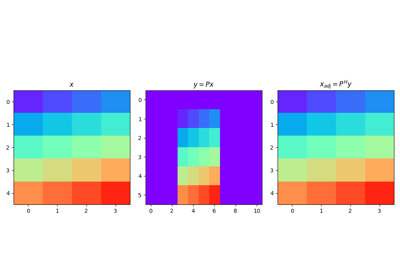class pylops.Pad(dims, pad, dtype='float64')[source]

Zero-pad model in forward model and extract non-zero subsequence in adjoint. Padding can be performed in one or multiple directions to any multi-dimensional input arrays.

Parameters: dims : Number of samples for each dimension pad : tuple Number of samples to pad. If dims is a scalar, pad is a single tuple (pad_in, pad_end). If dims is a tuple, pad is a tuple of tuples where each inner tuple contains the number of samples to pad in each dimension dtype : str, optional Type of elements in input array. ValueError If any element of pad is negative.

Notes

Given an array of size $$N$$, the Pad operator simply adds $$pad_{in}$$ at the start and $$pad_{end}$$ at the end in forward mode:

$y_{i} = x_{i-pad_{in}} \quad \forall i=pad_{in},pad_{in}+1,...,pad_{in}+N-1$

and $$y_i = 0 \quad \forall i=0,...,pad_{in}-1, pad_{in}+N-1,...,N+pad_{in}+pad_{end}$$

In adjoint mode, values from $$pad_{in}$$ to $$N-pad_{end}$$ are extracted from the data:

$x_{i} = y_{pad_{in}+i} \quad \forall i=0, N-1$
Attributes: shape : tuple Operator shape explicit : bool Operator contains a matrix that can be solved explicitly (True) or not (False)

Methods

 __init__(dims, pad[, dtype]) Initialize this LinearOperator. adjoint() Hermitian adjoint. apply_columns(cols) Apply subset of columns of operator cond([uselobpcg]) Condition number of linear operator. conj() Complex conjugate operator div(y[, niter]) Solve the linear problem $$\mathbf{y}=\mathbf{A}\mathbf{x}$$. dot(x) Matrix-matrix or matrix-vector multiplication. eigs([neigs, symmetric, niter, uselobpcg]) Most significant eigenvalues of linear operator. matmat(X) Matrix-matrix multiplication. matvec(x) Matrix-vector multiplication. rmatmat(X) Matrix-matrix multiplication. rmatvec(x) Adjoint matrix-vector multiplication. todense([backend]) Return dense matrix. toimag([forw, adj]) Imag operator toreal([forw, adj]) Real operator tosparse() Return sparse matrix. transpose() Transpose this linear operator.

## Examples using pylops.Pad¶